# Ncert Math Notes For Class 12 Relations and Functions Download PD

Ncert Math Notes For Class 12 Chapter 1 Relations And Functions Download PDf

## NCERT Notes Mathematics for Class 12 Chapter 1:- Relations and Functions

Let A and B be .two non-empty sets, then a function f from set A to set B is a rule whichassociates each element of A to a unique element of B.

It is represented as f: A → B and function is also called mapping.
f : A → B is called a real function, if A and B are subsets of R.

### Domain and Codomain of a Real Function

Domain and codomain of a function f is a set of all real numbers x for which f(x) is a real number. Here, set A is domain and set B is codomain.

### Range of a Real Function

Range of a real function, f is a set of values f(x) which it attains on the points of its domain.

### Classification of Real Functions

Real functions are generally classified under two categories algebraic functions and transcendental functions.

#### 1. Algebraic Functions

Some algebraic functions are given below
(i) Polynomial Functions If a function y = f(x) is given by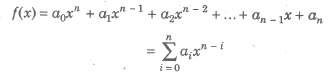where, a0, a1, a2,…, an are real numbers and n is any non -negative integer, then f (x) is called a polynomial function in x.

If a0 ≠ 0, then the degree of the polynomial f(x) is n. The domain of a polynomial function is the set of real number R.

e.g., y = f(x) = 3x5 – 4x2 – 2x +1

is a polynomial of degree 5.

(ii) Rational Functions If a function y = f(x) is given by f(x) = φ(x) / Ψ(x)

where, φ(x) and Ψ(x) are polynomial functions, then f(x) is called rational function in x.

(iii) Irrational Functions The algebraic functions containing one or more terms having nonintegral rational power x are called irrational functions.
e.g., y = f(x) = 2√x –3√x + 6

### 2. Transcendental Function

A. function, which is not algebraic, is called a transcendental function. Trigonometric, Inverse trigonometric, Exponential, Logarithmic, etc are transcendental functions.

##### Explicit and Implicit Functions

(i) Explicit Functions A function is said to be an explicit function, if it is expressed in the form y = f(x).

(ii) Implicit Functions A function is said to be an implicit function, if it is expressed in the form f(x, y) = C, where C is constant.
e.g., sin (x + y) – cos (x + y) = 2

#### Intervals of a Function

(i) The set of real numbers x, such that a ≤ x ≤ b is called a closed interval and denoted by [a, b] i.e., {x: x ∈ R, a ≤ x ≤ b}.

(ii) Set of real number x, such that a < x < b is called open interval and is denoted by (a, b) i.e., {x: x ∈ R, a < x < b}

(iii) Intervals [a,b) = {x: x ∈ R, a ≤ x ≤ b} and (a, b] = {x: x ≠ R, a < x ≤ b} are called semiopen and semi-closed intervals.

#### Graph of Real Functions

##### 1. Constant Function Let c be a fixed real number.

The function that associates to each real number x, this fixed number c is called a constant function i.e., y = f{x) = c for all x ∈ R.

Domain of f{x) = R
Range of f{x) = {c}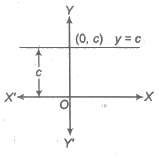##### 2. Identity Function

The function that associates to each real number x for the same number x, is called the identity function. i.e., y = f(x) = x, ∀ x ∈

R. Domain of f(x) = R
Range f(x) = R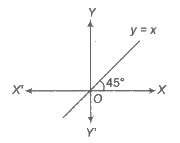##### 3. Linear Function

If a and b be fixed real numbers, then the linear function is defmed as y = f(x) = ax + b, where a and b are constants.

Domain of f(x) = R
Range of f(x) = R

The graph of a linear function is given in the following diagram, which is a straight line with slope a.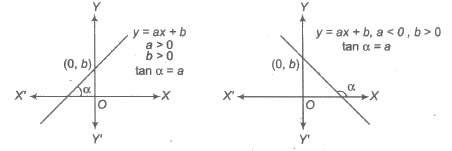If a, b and c are fixed real numbers, then the quadratic function is expressed as y = f(x) = ax2 + bx + c, a ≠ 0 ⇒ y = a (x + b / 2a)2 + 4ac – b2 / 4a

which is equation of a parabola in downward, if a < 0 and upward, if a > 0 and vertex at ( – b / 2a, 4ac – b2 / 4a).

Domain of f(x) = R

Range of f(x) is [ – ∞, 4ac – b2 / 4a], if a < 0 and [4ac – b2 / 4a, ∞], if a > 0 5. Square Root Function Square root function is defined by y = F(x) = √x, x ≥ 0.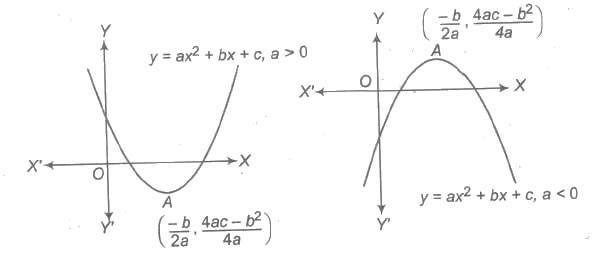##### 5. Square Root Function

Square root function is defined by y = F(x) = √x, x ≥ 0.

Domain of f(x) = [0, ∞)
Range of f(x) = [0, ∞)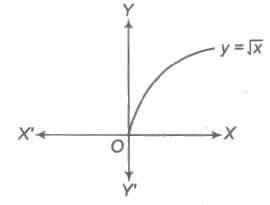##### 6. Exponential Function

Exponential function is given by y = f(x) = ax, where a > 0, a ≠ 1.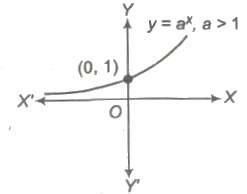##### 7. Logarithmic Function

A logarithmic function may be given by y = f(x) = loga x, where a > 0, a ≠ 1 and x > 0.

The graph of the function is as shown below. which is increasing, if a > 1 and decreasing, if 0 < a < 1.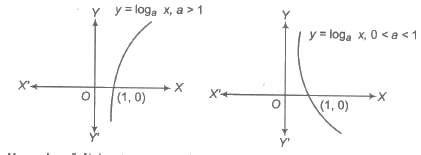Domain of f(x) = (0, ∞)
Range of f(x) = R

###### 8. Power Function

The power function is given by y = f(x) = xn ,n ∈ I,n≠ 1, 0. The domain and range of the graph y = f(x), is depend on n.

(a) If n is positive even integer.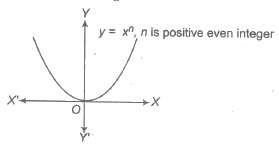i.e., f(x) = x2, x4 ,….

Domain of f(x) = R
Range of f(x) = [0, ∞)

(b) If n is positive odd integer.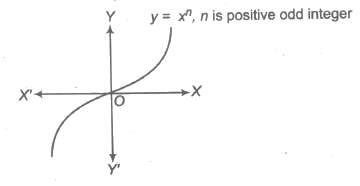i.e., f(x) = x3, x5 ,….

Domain of f(x) = R
Range of f(x) = R

(c) If n is negative even integer.

i.e., f(x) = x- 2, x – 4 ,….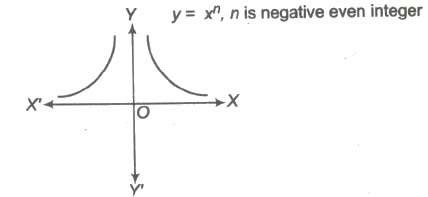Domain of f(x) = R – {0}
Range of f(x) = (0, ∞)

(d) If n is negative odd integer.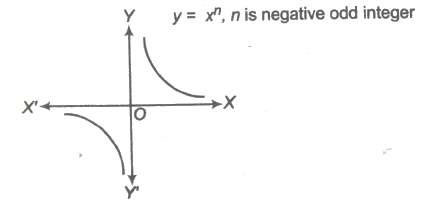i.e., f(x) = x- 1, x – 3 ,….

Domain of f(x) = R – {0}
Range of f(x) = R – {0}

###### 9. Modulus Function (Absolute Value Function)

Modulus function is given by y = f(x) = |x| , where |x| denotes the absolute value of x, that is

|x| = {x, if x ≥ 0, – x, if x < 0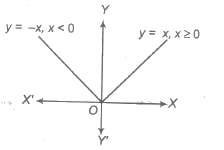Domain of f(x) = R
Range of f(x) = [0, &infi;)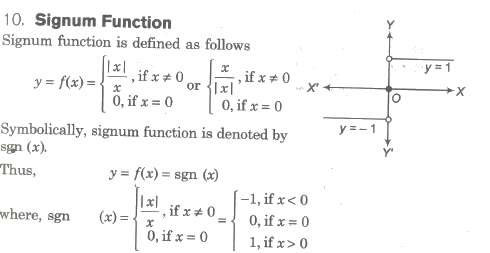Domain of f(x) = R
Range of f(x) = {-1, 0, 1}

###### 11. Greatest Integer Function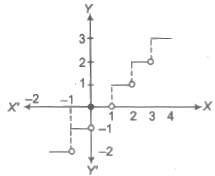The greatest integer function is defined as y = f(x) = [x]

where, [x] represents the greatest integer less than or equal to x. i.e., for any integer n, [x] = n, if n ≤ x < n + 1 Domain of f(x) = R Range of f(x) = I

#### Properties of Greatest Integer Function

(i) [x + n] = n + [x], n ∈ I
(ii) x = [x] + {x}, {x} denotes the fractional part of x.
(iii) [- x] = – [x], -x ∈ I
(iv) [- x] = – [x] – 1, x ∈ I
(v) [x] ≥ n ⇒ x ≥ n,n ∈ I
(vi) [x] > n ⇒ x ⇒ n+1, n ∈ I
(vii) [x] ≤ n ⇒ x < n + 1, n ∈ I
(viii) [x] < n ⇒ x < n, n ∈ I
(ix) [x + y] = [x] + [y + x – [x}] for all x, y ∈ R
(x) [x + y] ≥ [x] + [y]
(xi) [x] + [x + 1 / n] + [x + 2 / n] +…+ [x + n – 1 / n] = [nx], n ∈ N

##### 12. Least Integer Function

The least integer function which is greater than or equal to x and it is denoted by (x). Thus, (3.578) = 4, (0.87) = 1, (4) = 4, (- 8.239) = – 8, (- 0.7) = 0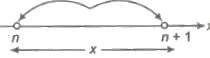In general, if n is an integer and x is any real number between n and (n + 1).
i.e., n < x ≤ n + 1, then (x) = n + 1

∴ f(x) = (x)

Domain of f = R
Range of f= [x] + 1

###### 13. Fractional Part Function

It is denoted as f(x) = {x} and defined as

(i) {x} = f, if x = n + f, where n ∈ I and 0 ≤ f < 1
(ii) {x} = x – [x]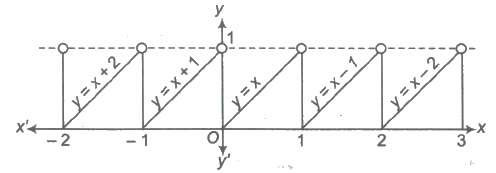i.e., {O.7} = 0.7, {3} = 0, { – 3.6} = 0.4
(iii) {x} = x, if 0 ≤ x ≤ 1
(iv) {x} = 0, if x ∈ I
(v) { – x} = 1 – {x}, if x ≠ I

#### Graph of Trigonometric Functions

##### 1. Graph of sin x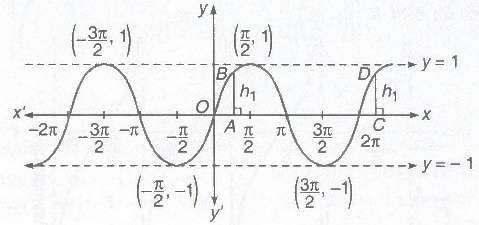(i) Domain = R
(ii) Range = [-1,1]
(iii) Period = 2π

###### 2. Graph of cos x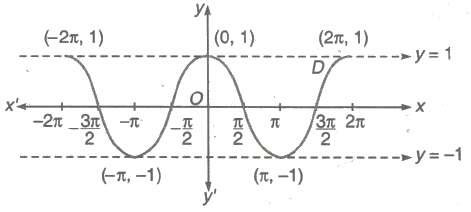(i) Domain = R
(ii) Range = [-1,1]
(iii) Period = 2π

###### 3.Graph of tan x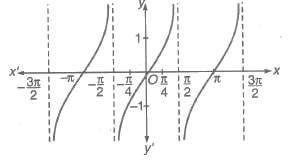(i) Domain = R ~ (2n + 1) π / 2, n ∈ I
(ii) Range = [- &infi;, &infi;]
(iii) Period = π

###### 4. Graph of cot x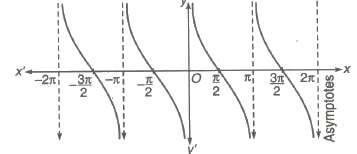(i) Domain = R ~ nπ, n ∈ I
(ii) Range = [- &infi;, &infi;]
(iii) Period = π

###### 5. Graph of sec x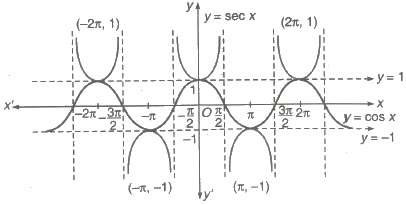(i) Domain = R ~ (2n + 1) π / 2, n ∈ I
(ii) Range = [- &infi;, 1] ∪ [1, &infi;)
(iii) Period = 2π

###### 6. Graph of cosec x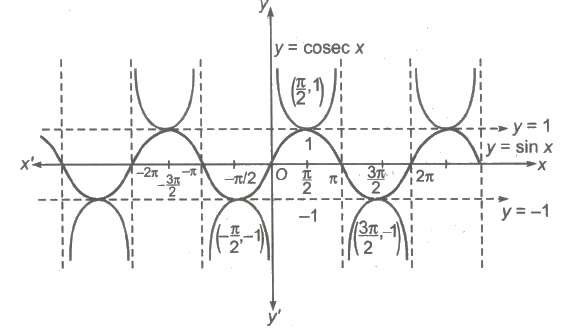(i) Domain = R ~ nπ, n ∈ I
(ii) Range = [- &infi;, – 1] ∪ [1, &infi;)
(iii) Period = 2π

##### Operations on Real Functions

Let f: x → R and g : X → R be two real functions, then

(i) Sum The sum of the functions f and g is defined as f + g : X → R such that (f + g) (x) = f(x) + g(x).
(ii) Product The product of the functions f and g is defined as fg : X → R, such that (fg) (x) = f(x) g(x) Clearly, f + g and fg are defined only, if f and g have the same domain. In case, the domain of f and g are different. Then, Domain of f + g or fg = Domain of f ∩ Domain of g.
(iii) Multiplication by a Number Let f : X → R be a function and let e be a real number .
Then, we define cf: X → R, such that (cf) (x) = cf (x), ∀ x ∈ X.
(iv) Composition (Function of Function) Let f : A → B and g : B → C be two functions. We define gof : A → C, such that got (c) = g(f(x)), ∀ x ∈ A
Alternate There exists Y ∈ B, such that if f(x) = y and g(y) = z, then got (x) = z

###### Periodic Functions
A function f(x) is said to be a periodic function of x, provided there exists a real number T > 0, such that F(T + x) = f(x), ∀ x ∈ R

The smallest positive real number T, satisfying the above condition is known as the period or the fundamental period of f(x) ..
##### Testing the Periodicity of a Function

(i) Put f(T + x) = f(x) and solve this equation to find the positive values of T independent of x.
(ii) If no positive value of T independent of x is obtained, then f(x) is a non-periodic function.
(iii) If positive val~es ofT independent of x are obtained, then f(x) is a periodic function and the least positive value of T is the period of the function f(x).

##### Important Points to be Remembered

(i) Constant function is periodic with no fundamental period.
(ii) If f(x) is periodic with period T, then 1 / f(x) and. √f(x) are also periodic with f(x) same period T.
{iii} If f(x) is periodic with period T1 and g(x) is periodic with period T2, then f(x) + g(x) is periodic with period equal to LCM of T1 and T2, provided there is no positive k, such that f(k + x) = g(x) and g(k + x) = f(x).
(iv) If f(x) is periodic with period T, then kf (ax + b) is periodic with period T / |a|’ where a, b ,k ∈ R and a, k ≠ 0.
(v) sin x, cos x, sec x and cosec x are periodic functions with period 2π.
(vi) tan x and cot x are periodic functions with period π.
(vii) |sin x|, |cos x|, |tan x|, |cot x|, |sec x| and |cosec x| are periodic functions with period π.
(viii) sinn x, cosn x, secn x and cosecnx are periodic functions with period 2π when n is odd, or π when n is even .
(ix) tann x and cotnx are periodic functions with period π.
(x) |sin x| + |cos x|, |tan x| + |cot x| and |sec x| + |cosec x| are periodic with period π / 2.

##### Even and Odd Functions

Even Functions A real function f(x) is an even function, if f( -x) = f(x).
Odd Functions A real function f(x) is an odd function, if f( -x) = – f(x).

###### Properties of Even and Odd Functions

(i) Even function ± Even function = Even function.
(ii) Odd function ± Odd function = Odd function.
(iii) Even function * Odd function = Odd function.
(iv) Even function * Even function = Even function.
(v) Odd function * Odd function = Even function.
(vi) gof or fog is even, if anyone of f and g or both are even.
(vii) gof or fog is odd, if both of f and g are odd.
(viii) If f(x) is an even function, then d / dx f(x) or ∫ f(x) dx is odd and if dx .. f(x) is an odd function, then d / dx f(x) or ∫ f(x) dx is even.
(ix) The graph of an even function is symmetrical about Y-axis.
(x) The graph of an odd function is symmetrical about origin or symmetrical in opposite quadrants.
(xi) An even function can never be one-one, however an odd function mayor may not be oneone.

#### Different Types of Functions (Mappings)

##### 1. One-One and Many-One Function

The mapping f: A → B is a called one-one function, if different elements in A have different images in B. Such a mapping is known as injective function or an injection.

###### Methods to Test One-One

(i) Analytically If x1, x2 ∈ A, then f(x1) = f(x2) => x1 = x2 or equivalently x1 ≠ x2 => f(x1) ≠ f(x2)
(ii) Graphically If any .line parallel to x-axis cuts the graph of the function atmost at one point, then the function is one-one.
(iii) Monotonically Any function, which is entirely increasing or decreasing in whole domain, then f(x) is one-one.
Number of One-One Functions Let f : A → B be a function, such that A and B are finite sets having m and n elements respectively, (where, n > m).
The number of one-one functions n(n – 1)(n – 2) …(n – m + 1) = { nPm, n ≥ m, 0, n < m
The function f : A → B is called many – one function, if two or more than two different elements in A have the same image in B.

##### 2. Onto (Surjective) and Into Function

If the function f: A → B is such that each element in B (codomain) is the image of atleast one element of A, then we say that f is a function of A ‘onto’ B.
Thus, f: A → B, such that f(A) = i.e., Range = Codomain Note Every polynomial function f: R → R of degree odd is onto.
Number of Onto (surjective) Functions Let A and B are finite sets having m and n elements respectively, such that 1 ≤ n ≤ m, then number of onto (surjective) functions from A to B is nΣr = 1 (- 1)n – r nCr rm = Coefficient ofn in n! (ex – 1)r If f : A → B is such that there exists atleast one element in codomain which is not the image of
Thus, f : A → B, such that f(A) ⊂ B
i.e., Range ⊂ Codomain

###### Important Points to be Remembered

(i) If f and g are injective, then fog and gof are injective.
(ii) If f and g are surjective, then fog is surjective.
(iii) Iff and g are bijective, then fog is bijective.

###### Inverse of a Function

Let f : A → B is a bijective function, i.e., it is one-one and onto function.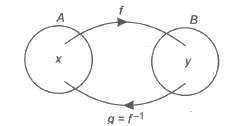We define g : B → A, such that f(x) = y => g(y) = x, g is called inverse of f and vice-versa.
Symbolically, we write g = f-1
Thus, f(x) = y => f-1(y) = x

### NCERT Books Free Pdf Download for Class 5, 6, 7, 8, 9, 10 , 11, 12 Hindi and English Medium

 Mathematics Biology Psychology Chemistry English Economics Sociology Hindi Business Studies Geography Science Political Science Statistics Physics Accountancy